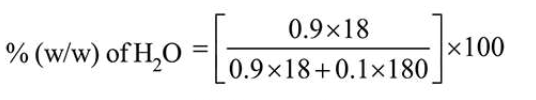# The mole fraction of glucoseQuestion:

The mole fraction of glucose $\left(\mathrm{C}_{6} \mathrm{H}_{12} \mathrm{O}_{6}\right)$ in an aqueous binary solution is $0.1$. The mass percentage of water in it, to the nearest integer, is ___________ .

Solution:

(47)

Let total mole of solution $=1$

So, mole of glucose $=0.1$

Mole of $\mathrm{H}_{2} \mathrm{O}=0.9$$=47.368=47.37$